# Brain Teaser :: Can You Solve the LOGIC Triangle Puzzle?

in STEMGeeks3 months ago

Hey All; @StemGeeks Mathematician;

Without further, ado, let's get to our Brain Teaser :: Can You Solve the LOGIC Triangle Puzzle?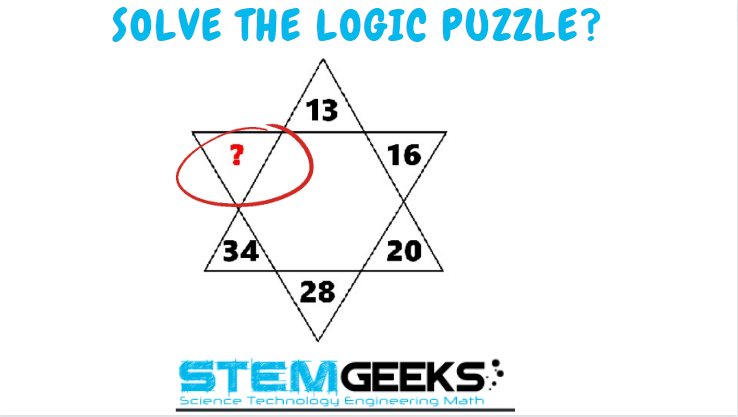Have a close look at the image given and see if you can find the missing number in the upside TRAINGLE. Just remember there hasn't have to be a logic always. The puzzle isn't that hard to solve.

There are few different solution and simple basic math's knowledge is required to get the equation right. Just give it a try - all you need to do is pick up a rough paper and see the equation that's being formed and you will get the answer. Just give it a try and its that simple I assure you. There is no right or wrong - comment your answer on the reply box below to enter a chance to WIN 10 STEM tokens...

### STEM token GiveAway...

I'll be again doing a giveaway of STEM tokens to the lucky random winner with the correct answer. For the last contest, which was Brain Teaser :: Can You Find the Missing Number?.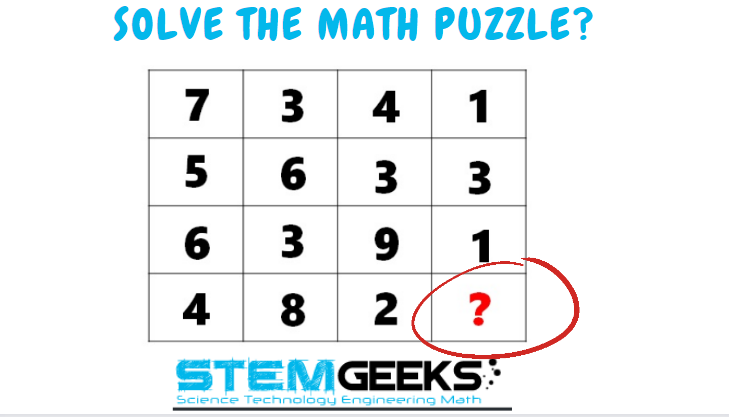### Solution :: Brain Teaser :: Can You Find the Missing Number?

###### Pattern being followed was:: sum the total of each rows to see the pattern being made i.e. ::
• First Row Adding Up - 7 + 3 + 4 + 1 = 15
• Second Row Adding Up - 5 + 6 + 3 + 3 = 17
• Third Row Adding Up - 6 + 3 + 9 + 1 = 19

The final row in question:: if you see every rows last number is an increment of 2; therefore for the last row in question we have::

• Final Row :: 4 + 8 + 2 + ? = 21

• 4 + 8 + 2 + 7 = 21

Finally the answer to the puzzle was:: 7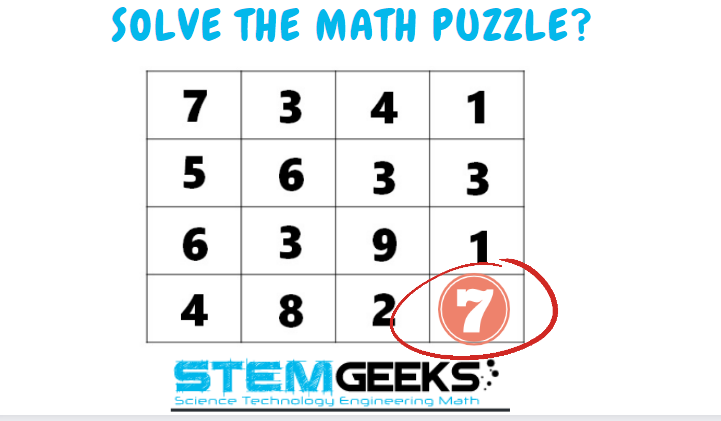We had TWO entries and only of them provided the right answer. Hence the Winner is :: @sperosamuel15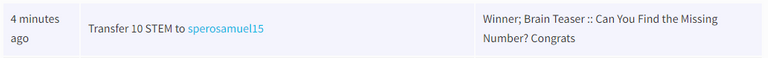Congratulations - You WIN yourself 10 STEM tokens each. You should be having the rewards in your STEM Wallet Soon.

### Math Quote for the Day::

Here is the motivation to solve the Math Puzzle?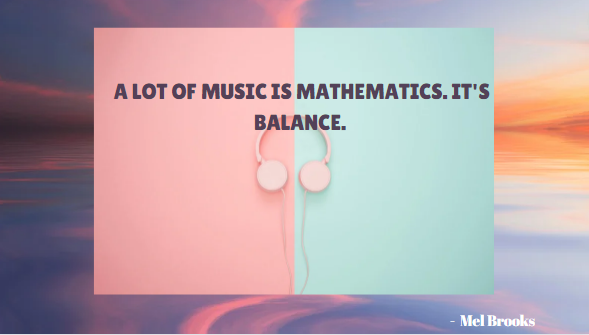If you like my work, then please spread the Word.. that we do have the Math Brain Teasers competition here @StemGeeks platform. Reblog is much appreciated.

Best RegardsFind Me on the Other Social Media Platforms::PS:: All the Maths Brain Teasers; are made by me using the Pro Canva License Version

Sort:

Thank you very much for the rewards.... I appreciate

Congratulations, @gungunkrishu Your Post Got 81.29% Boost.
@gungunkrishu Burnt 75 UPME & We Followed That Lead.

##42

Logic behind:

• 13*2 = 26 + 2 = 28
• 16*2 = 32+ 2 = 34
• 20*2 = 40 + 2 = 42Well done @gungunkrishu! You successfully guessed the match result and unlocked your badge!Click on the badge to see your board.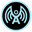@arcange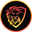@leofinance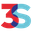@threespeak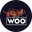@wrestorgonlineWell done @gungunkrishu! You successfully guessed the match result and unlocked your badge!Click on the badge to see your board.@arcange@leofinance@threespeak@wrestorgonline

Logic
The sum of equilateral triangles are equal, hence, if triangle A has

13+20+34= 67
Then, Triangle B should equal 67

16+28+?= 67
44+? = 67
?= 67-44
?= 23
Hence, the missing number to make the sum of triangle B 67 is 23

The correct answer is 42. The most common element there is the multiplication and addition of 2 to the numbers. So we have:

13 x 2 + 2 = 28
16 x 2 + 2 = 34
20 x 2 + 2 = 42

@rimurutempest got it earlier than me. @gungunkrishu

3 months ago Reveal Comment